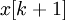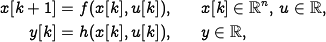# Errata: In Exercise 5.8d, the left hand side of the first equation should be x(k+1)

In Exercise 5.8, part d, the left hand side of the state update equation should be. The correct equation is